## Connections and interpretations

### Reinforcement learning¶

• states defined via features
• the agent is a classifier
• rewards?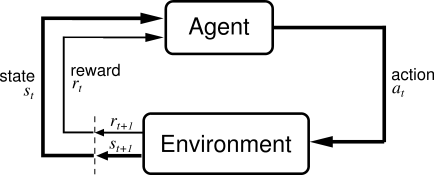Inverse reinforcement learning

• we have the expert policy (inferred from the gold standard in the training data)
• we infer the per-action reward function (rollin/out)

LOLS with classifier only rollouts is RL (Chang et al., 2015)

### Bandit learning¶

Rolling out each action can be expensive:

• many possible actions
• expensive loss functions to calculate

What if we only rollout one?Bandit Learning

Structured Contextual Bandit LOLS (Chang et al., 2015)

Sokolov et al. (2016) used bandit feedback for chunking

### Semi/Unsupervised learning¶

Learning with non-decomposable loss functions means

• no need to know the correct actions
• learn to predict them in order to minimize the loss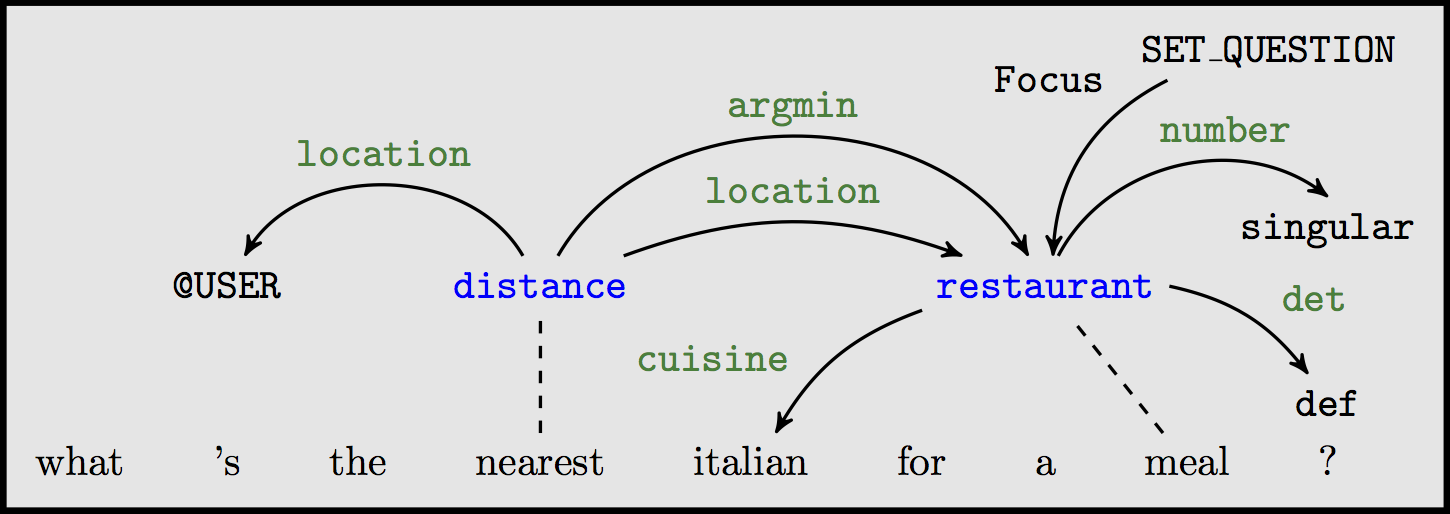UNSEARN (Daumé III, 2009): Predict the structured output so that you can predict the input from it (auto-encoder!)

### Negative data sampling¶

In :
paths = [[],[(0,4),(1,3)],[(0,4),(1,3),(2,2)],[(0,4),(1,3),(2,2),(3,1)]]
rows = ['Noun', 'Verb', 'Modal', 'Pronoun','NULL']
columns = ['NULL','I', 'can', 'fly']
gold_path = [(0,4),(1,3),(2,2),(3,1)]
cbs=[]
cb_gold = cg.draw_cost_breakdown(rows, columns, gold_path)
cbs.append(cb_gold)
wrong_path = [(0,4),(1,2)]
cb_wrong = cg.draw_cost_breakdown(rows, columns, wrong_path)
cbs.append(cb_wrong)
p = gold_path.copy()
for i in range(4):
p = gold_path.copy()
p = (gold_path,i)
if p == gold_path:
cost = 0
elif i==1:
cost =2
p = (3,0)
else:
cost = 1
cbs.append(cg.draw_cost_breakdown(rows, columns, p, cost, p, roll_in_cell=p,roll_out_cell=(3,0), explore_cell=p))
util.Carousel(cbs)

Out:
• Expert action sequence → positive example
• All other action sequences → negative examples

Generate useful negative samples around the expert, related to adversarial training (Ho and Ermon, 2016) )

### Coaching¶

If the optimal action is difficult to
predict, the coach teaches a good one
that is easier (He et al., 2012)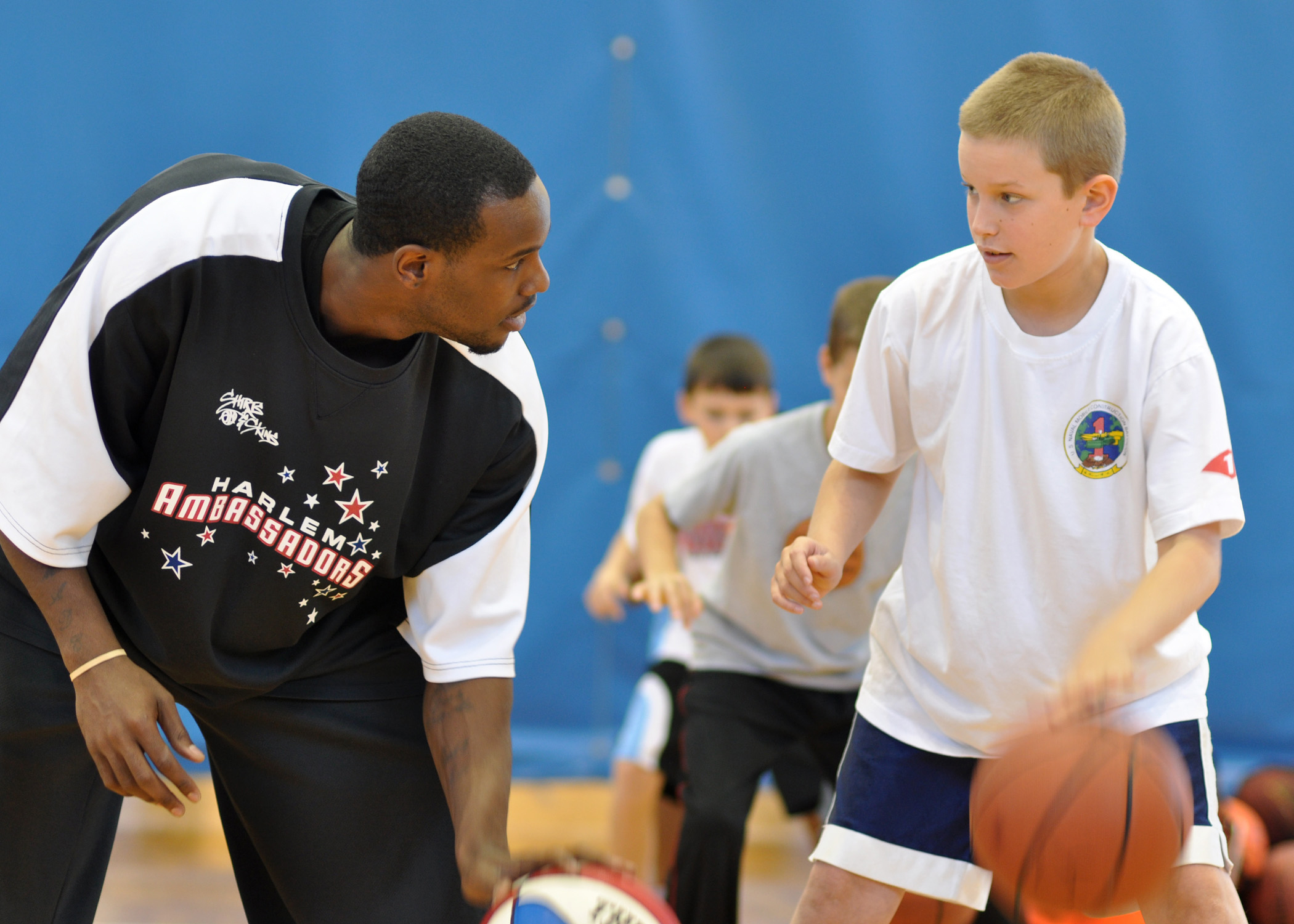Expert: $\alpha^{\star}= \mathop{\arg \min}_{\alpha \in {\cal A}} L(S_t(\alpha,\pi^{\star}),\mathbf{y})$

Coach: $\alpha^{\dagger}= \mathop{\arg \min}_{\alpha \in {\cal A}} \lbrace L(S_t(\alpha,\pi^{\star}),\mathbf{y}) - \lambda \cdot f(\alpha, \mathbf{x}, S_t)\rbrace$

i.e. $\alpha^{\dagger}=\mathop{\arg \min}_{\alpha \in {\cal A}} \lbrace loss(\alpha) - \lambda \cdot classifier(\alpha) \rbrace$

### Changeprop¶

Speed-up action costing in LOLS (Viera and Eisner, 2016):

If one action changes, don't assess the cost of the whole trajectory (under assumptions), propagate the change!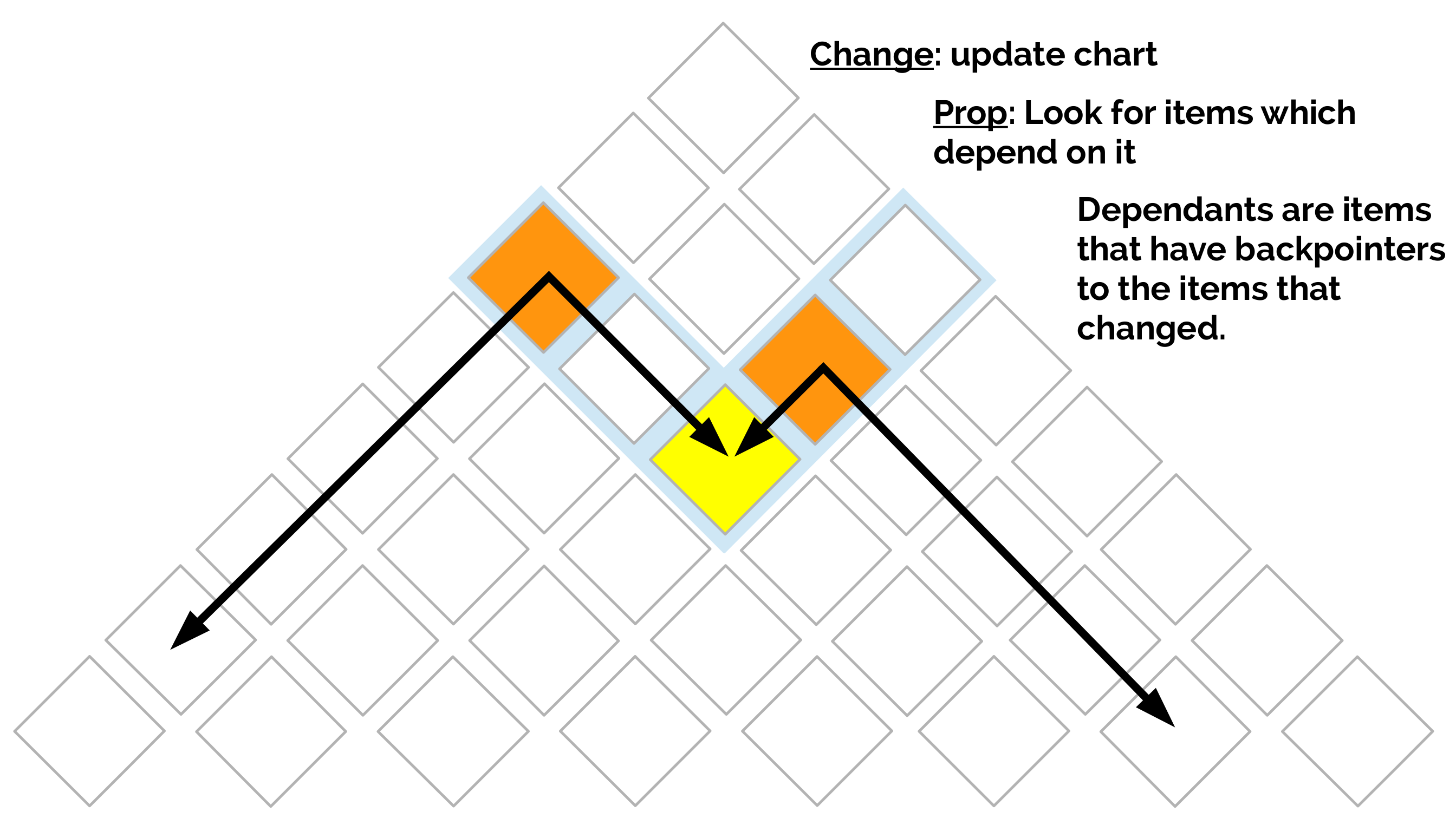</a>

### What about Recurrent Neural Networks?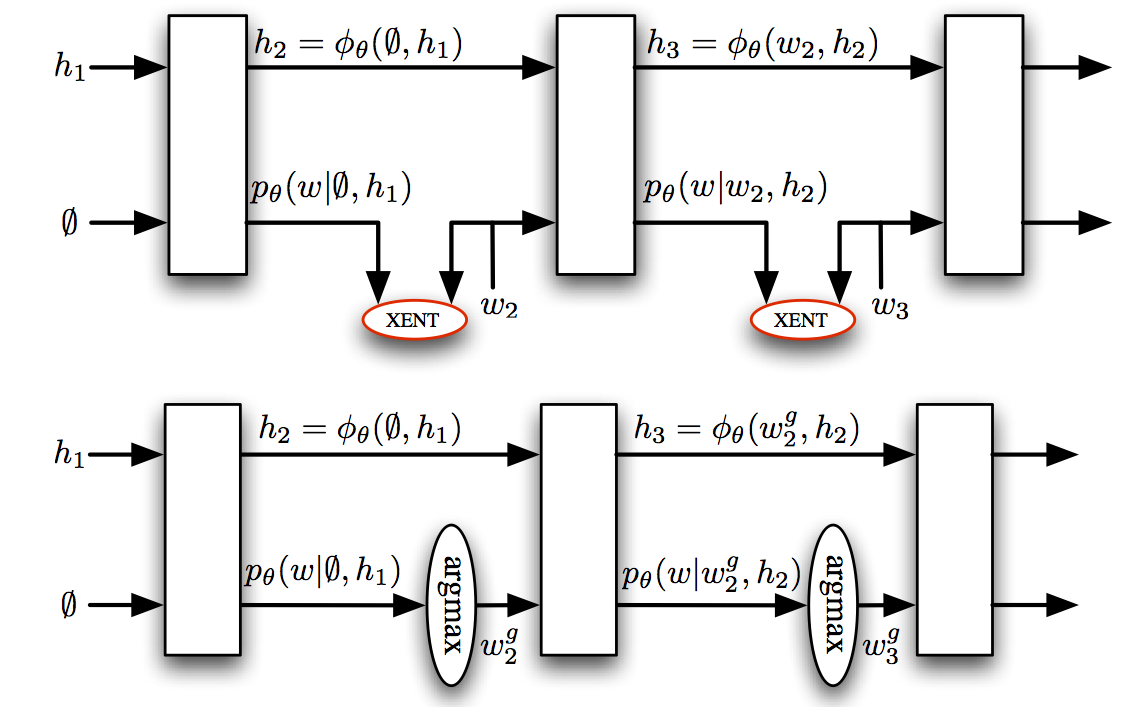They face similar problems:

• trained at the word rather than sentence level
• assume previous predictions are correct

### Imitation learning and RNNs¶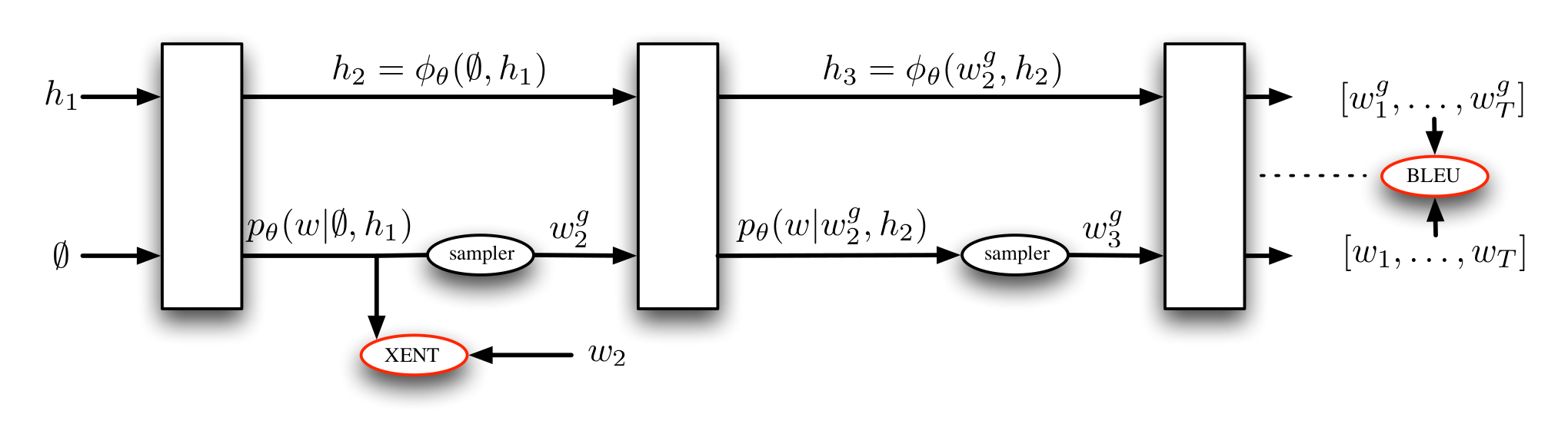• DAgger mixed rollins, similar to scheduled sampling (Bengio et al., 2015)
• MIXER (Ranzato et al., 2016): Mix REINFORCE-ment learning with imitation: we have the expert policy!
• no rollouts, learn a regressor to estimate action costs
• end-to-end back propagation through the sequence

### Actor-critic¶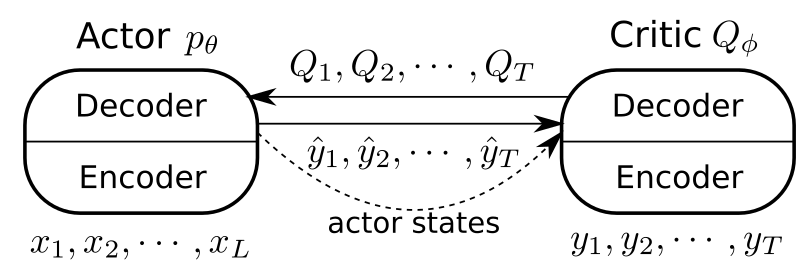• actor: the RNN we are learning to use during testing
• critic: another RNN that is trained to predict the value of the actions of the critic

### Summary so far¶

• basic concepts
• loss function decomposability
• expert policy
• imitation learning
• rollin/outs
• DAgger algorithm
• DAgger with rollouts and LoLS
• connections and interpretations

### After the break¶

• Applications:
• dependency parsing
• natural language generation
• semantic parsing
• Practical advice
• making things faster
• debugging